# Learn the concept of Arrays (Lists) in Python

0
6028In our Part 7 of Python series we learned about different types of functions in Python, today in this tutorial we will learn the concepts of Arrays (Lists) in Python. So let’s get started.

What are Lists?

A list (array) is a set of objects. Individual objects can be accessed using ordered indexes that represent the position of each object within the list.

Why Lists?

Your manager asked you to write a Python program that accepts some numbers from the user. Once entered, the numbers should be stored someway. Then the stored numbers should be sorted in ascending and descending orders, and printed to the user in both cases.

Ah, and the most important requirement is that the program should keep asking the user for input until the user enters -99999. At this point the program stops prompting for new numbers, starts processing, and finally prints the sorted numbers. This means the number of the user’s input numbers is variable.

Now, the big question: How would you achieve this requirement? How could you store a variable number of inputs (which you don’t know in advance)?

Lists will give us the answer, soon.

* * * * * *

The following is a list containing four elements:

`myarray = [3, 7, -3, 12]`

To read the content of the first element, and assign it to the variable x:

`x = myarray`

To set the value of the fourth (last) element to 0:

`myarray = 0`

Note

To access the first element of an array, the element at index 0 is referenced; myarray. To access the second element, the element at index 1 is referenced; myarray, and so on. As a general rule, to access the nth element of an array, refer to the element at index n-1.

* * * * * *

Let’s get more familiar with lists by taking some examples.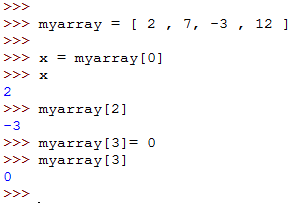The len() function
The len() function takes a list as an argument, and returns the length of the list.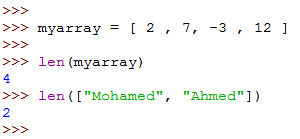The list named myarray contains four elements, so the output of len(myarray) is 4.

Accessing the last element in a list
To access the last element in an array, we can do it using either of two ways:

• Using the array length: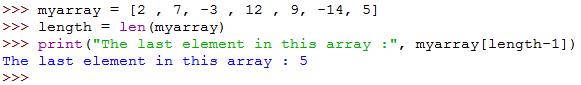• Using the Negative index: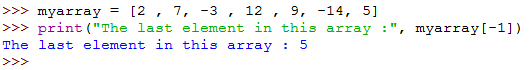So, myarray[-1] is exactly equivalent to myarray[length(myarray) – 1]
Both methods have the same effect, so you are free to use either one.

* * * * * *

Array Slicing
Slicing can be defined as taking a subset of an existing array to create a new one.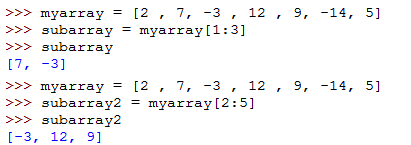When we say subarray = myarray[1:3] , we are asking the interpreter to declare a new array called subarray, and initialize it with values from myarray. This is exactly equivalent to:

```subarray = myarray
subarray = myarray
```

Passing Lists as arguments to functions

Lists can be passed to functions as arguments, just like ordinary variables. We have already tried this when we talk about the len() function.

To simplify the idea, consider the following script that declares a function avg_list that takes a list as its argument, and returns the average of the list.

• First the function is defined using the def statement, with one argument specified.
• The variable that will store the (accumulative) total is initialized to 0.
• A for loop is needed to loop on the elements of the passed list (mylist). So, the loop variable i will take all the possible values from the range 0 up to the length of the array mylist (excluding the upper value: len(mylist)) .
• The elements of the array mylist are referenced one after another (using the loop variable as index). In each iteration, the value of the element is added to the variable total.
• At last the average is calculated and returned to the calling statement.

Simple and logic, huh!!

* * * * * *

Getting Minimum and Maximum values in a list
To get the element having the minimum value in a list, use the min() function: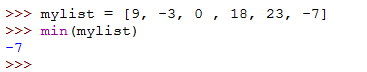Similarly, to get the element having the maximum value in list, use the max() function: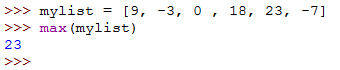Appending to Lists
There will be cases (like the one we will discuss soon) that you will need to add (append) a new element to an existing array. To do this, use the list.append() method: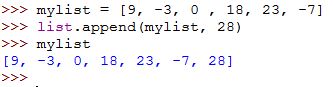Sorting Lists
A list can be sorted using the sort() method: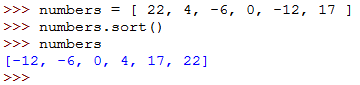Reversing Lists
A list can have its elements reversed using the reverse() method: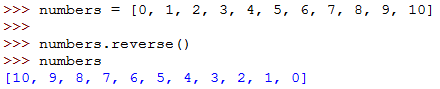* * * * * *

Example

Back to the example I gave you earlier in this article under section Why Lists.
Now, and after gaining some fair knowledge about lists, have you had an idea on how to write the required program?

Check the following script: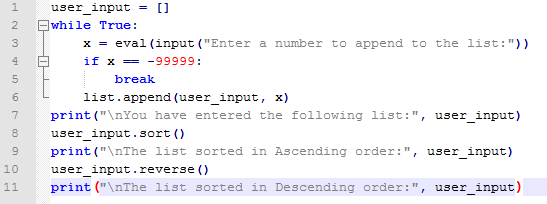Let’s execute it, and see what will happen: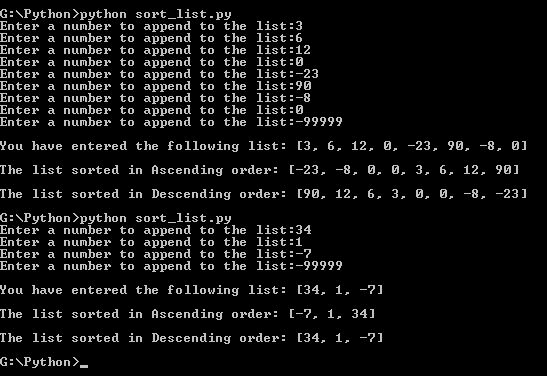Have you seen that?! It worked!!!

Now, to the explanation:

• Line 1: declares the empty list user_input.
• Line 2: starts an infinite loop.
• Lines 3-6 : the loop body:
• Line 3: prompts the user to input a number. After being evaluated, the number is assigned to the variable x.
• Line 4: checks if the input equals -99999 (the exit condition). If so, Line 5 executes the break statement that exits the loop.
• Line 6: if the input doesn’t meet the exit condition, this line is executed. It appends the input number to the list user_input.
• Line 7: prints the resulting list of numbers user_input.
• Line 8: uses the sort() method to restructure the user_input array, by sorting its elements in Ascending order.
• Line 9: prints the sorted list.
• Line 10: reverses the order of list (which has been sorted in ascending order). This reverses the order to be Descending.
• Line 11: prints the reversed list.

Simple, logic, and straightforward, isn’t it?!

* * * * * *

In this article, we have tackled a very important concept: Lists. Lists are useful for storing and processing related collections of data.

See you in another series, and another topic.